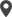Enable Dark Mode!By: Sruthi C Nair

# How to Use Python Math Module | Python Arithmetic Operators

## Technical

Sometimes necessary to implement mathematical calculations in some types of projects like mathematical or scientific projects. Python provides such calculations provide the math module in python. All advanced operations like trigonometric, logarithmic, and also essential operations such as multiplication(*),  division(/), subtraction(-), addition(+), and exponential functions are provided by the Math module.
The built_in functions in the math module provide to find the values and degrees of cosine sine and values of any angle. There is some function such as tan() , cos(),tan(). Let's see the use of those functions
`import mathb =10print(math.cos(b)print(math.sin(b)print(math.tan(b)`
These are returned with corresponding sine, cosine, and tangent values. Also, we can convert radians to degrees and vice versa.
math.degrees(b)
If you need any gamma values, you can simply use like,
math.gamma(variable)
Math module Constants
* math. e return Euler’s Number
* math. pi return pi value.
* math. the ratio of the perimeter to the radius of a circle in math is the return value
* math. Nan returns not a number value
* math.inf returns positive infinity floating point value
* math. fabs(x)returns the real number where x is a real number
Ceiling and Floor
math. ceil () is used to round the nearest integer a number Up to
math. floor() is used  returns the integer which is smaller than the number itself, and  also takes a number with decimal as an argument
`n=5.8math.ceil(n)math.floor(n)Output32`
How to find the factorial of a number?
Simply we can use math.factorial(number)
`math.factorial(6)Output720`
Can we find the common divisor of two numbers? Such cases deal with math. gcd() function.
math.gcd(num1,num2)
a) To Find the absolute value of a number, we can use math.fabs()
math. fabs(-5), we get the output as 5.0
b) Also math.pow () returns the power of the number
c) returns the math. sqrt() is  the square root of the number
Sum of Iterables
The math. sum() is the easiest way to find the sum of the values of an iterable, that is, the Sum of Iterables, without using a loop. In this situation, the function gives the sum of the values. We can use such arrays, tuples, or lists as iterable input
print(math.fsum([11, 22, 33, 44, 55]))
Truncate Numbers with trunc()
When you need to remove the decimal part and only keep the integer part of a number, then we can choose the function trunc().
math.trunc(895.5)
Find closeness of numbers using isclose()
Using isclose(), you can determine two numbers that are close to each other. So it returns true and false value
math.isclose(7,8)
Also, the output of this function is false
math.isclose(7.99999,8) return true value
We can use the Python math module to deal with mathematical operations. To perform useful and common mathematical calculations within our application, we can use The Python math module.

If you need any assistance in odoo, we are online, please chat with us.

### Recent Posts

#### How to Transfer Cash Between Accounts in Odoo 16 AccountingCalicut

Cybrosys Technologies Pvt. Ltd.
Neospace, Kinfra Techno Park
Kakkancherry, Calicut
Kerala, India - 673635London

Cybrosys Limited
Alpha House,
100 Borough High Street, London,
SE1 1LB, United KingdomKochi

Cybrosys Technologies Pvt. Ltd.
1st Floor, Thapasya Building,
Kochi, India - 682030.Bangalore

Cybrosys Techno Solutions
The Estate, 8th Floor,